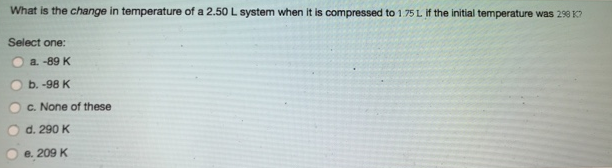# Problem: What is the change in temperature of a 2.50 L system when it is compressed to 1.75 L. If the initial temperature was 299 K? Select one: a. -89 K b. -98 K c. None of these d. 290 K e. 209 K

###### FREE Expert Solution
99% (166 ratings)###### Problem Details

What is the change in temperature of a 2.50 L system when it is compressed to 1.75 L. If the initial temperature was 299 K?

Select one:

a. -89 K

b. -98 K

c. None of these

d. 290 K

e. 209 K